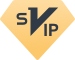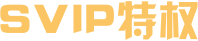￥36.9元起
38.9 拼团价

## 【开心哈乐儿童乐园·康营易事达店】拼团38.9元起抢全天畅玩，欢乐淘气堡、益智游戏区、刺激蹦床场...溜娃打卡必备地！！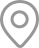北京市朝阳区香江北路康营易事达商场二层东侧2023-04-26 23:59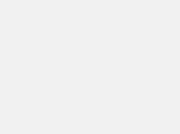【售卖日期】

【使用日期】

【营业时间】

10:00-21:00；

【活动价格】

39.9元,原价￥138，一大一小全天畅玩平日票(周一至周五)

49.9元,原价￥198一大一小全天畅玩票(平日周末节假日通用)

99.9元,原价￥238一大一小通用门票+75币（币有效期30天）

*单加儿童需要全价票一张，单加成人20元陪同票。

【费用包含】

【咨询电话】

13651397052

【换票地址】

【活动地址】

【购买须知】

1. 禁止外带食品饮料，孩子需要喝水请到休息区饮用

2.如遇大客流，内场人满，请排队等待入场，或更改其他时间游玩。

3. 无需预约，周末节假日通用

4. 进场家长需穿袜子(自备)，现场购买5元

5、 酒后、高血压、心脏病、及妇及所有心血管疾病、呼吸道疾病、外伤、眩晕症患者，请勿入场。

6. 请勿在没有基础和自我保护的能力下做任何危险动作、高难度翻转动作，例如手翻、空翻、旋转等。

7. 如果需要休息，不要在蹦床上停留，一个格子仅限一个人跳，这样安全比较有保障。

【备注】有效期内未验证使用可退，特价产品过期不退不换<<<<<<<<<

童趣满分夏日绽放

<<<<<<<<<<<<<<<<<<

童趣满分夏日绽放

随着春天脚步的逼近

<<<<<<<<<<<<<<<<<<

<<<<<<<<<<<<<<<<<<

<<<<<<<<<<<<<<<<<<

<<<<<<<<<<<<<<<<<<

<<<<<<<<<<<<<<<<<<

<<<<<<<<<<<<<<<<<<

<<<<<<<<<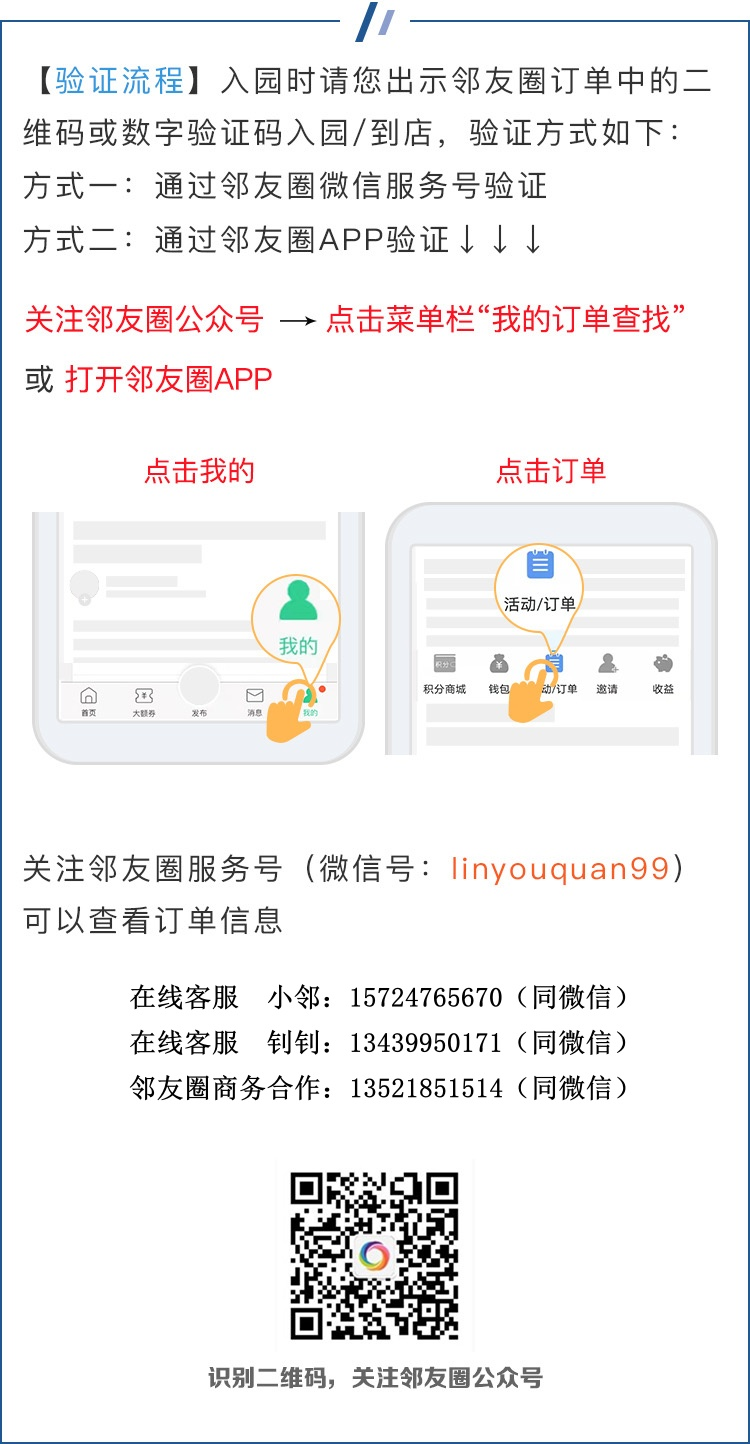￥799邻友圈·发现周边更多好活动

【开心哈乐儿童乐园·康营易事达店】拼团38.9元起抢全天畅玩，欢乐淘气堡、益智游戏区、刺激蹦床场...溜娃打卡必备地！！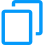复制推广文案